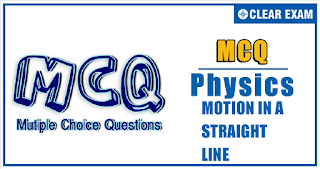## Physics Quiz-4

If an object changes its position with respect to its surroundings with time, then it is called in motion. It is a change in the position of an object over time. Motion in a straight line is nothing but linear motion. As the name suggests, it’s in a particular straight line, thus it can be said that it uses only one dimension.

Q1. If a particle travels n equal distance with speeds v_1,v_2,…,v_n, then the average speed V ̅ of the particle will  be such that
Solution
t_1=s/v_1 ,t_2=s/v_2 ,…,t_n=s/v_n
Average speed =(Total distance)/(Total time)

V ̅=ns/(t_1+t_2+...+t_n )
ns/(s/v_1 +s/v_2 +...+s/v_n )=n/(1/v_1 +1/v_2 +...+1/v_n )
Taking reciprocal, we get 1/V ̅ =1/n (1/v_1 +1/v_2 +...+1/v_n )

Q2.The location of a particle is changed. What can we say about the displacement and distance covered by the particle?
•  Both cannot be zero
•  One of the two may be zero
•  Both must be zero
•  Both must be equal
Solution
If the location of a particle changes, then both distance and displacement must have some value.

Q3.  A ball is released from the top of a tower of height h. It takes time T to reach the ground. What is the position of the ball (from ground) after time T/3?
•  h/9 m
•  7h/9 m
•  8h/9 m
•  17h/18 m
Solution
We have h=1/2 gT^2
In T/3 second, distance fallen =1/2 g(T/3)^2=h/9
So position of the ball from the ground is
h-h/9=8h/9 m

Q4. The displacement-time graph of a body is shown in Fig

The velocity-time graph of the motion of the body will be
Solution
From 0 to t_1, velocity  is positive and constant as indicated by positive and constant slope
From t_1 to t_3, slope is zero, hence velocity is zero
From t_3 to t_4, velocity is negative and constant as indicated by negative and constant as indicated by negative and constant slope
Option (d) satisfies all these observations

Q5.The magnitude of displacement is equal to the distance covered in a given interval of time if the particle

•  Moves with constant acceleration of time if the particle
•  Moves with constant speed
•  Moves in same direction with constant velocity or with variable velocity
•  Moves with constant velocity
Solution
To have distance equal to magnitude of displacement, the particle has to move in the same direction. The velocity may or may not be constant.

Q6.
 A thief is running away on a straight road in a jeep moving with a speed of 9 ms^(-1). A                       policeman chases him on a motor cycle moving at a speed of 10 ms^(-1). If the instantaneous         separation of the jeep from the motor cycle is 100 m, how long will it take for the policeman             to catch the thief?
•  1 s
•  19 s
• 90 s
•  100 s
Solution
Relative velocity of policeman w.r.t. the thief is 10-9=1 ms^(-1). Since the relative separation between them is 100 m, the time taken will be = relative separation relative velocity =100/1 = 100 s

Q7.
The numerical value of the ratio of instantaneous velocity to instantaneous speed is
•  Always less than one
•  Always equal  to one
•  Always more than one
•  Equal to or less than one
Solution
At any instant, the magnitudes of velocity and speed will be equal

Q8. A body is thrown vertically upwards from A, the top of a tower. It reaches the ground in time             t_1. If it is thrown vertically downwards from A with the same speed, it reaches the ground in           time t_2. If it is allowed to fall freely from A, then the time it takes to reach the ground is given         by

•  t=(t_1+t_2)/2
•  t=(t_1-t_2)/2
•  t=(t_1-t_2)/2
•  t=√(t_1/t_2)

Solution
Suppose the body be projected vertically upwards from A with a speed u_0
Using equation s=ut+(1/2)at^2, we get
For first case: -h=u_0 t_1-(1/2)gt_1^2  (i)
For second case: -h=-u_0 t_2-(1/2)gt_2^2  (ii)
(i)—(ii) ⇒0=u_0 (t_2+t_1 )+(1/2)g(t_2^2-t_1^2)
⇒u_0=(1/2)g(t_1-t_2)  (iii)
Putting the value of u_0 in (ii), we get
-h=-(1/2)g(t_1-t_2 ) t_2-(1/2)gt_2^2
⇒h=1/2 gt_1 t_2      (iv)
For third case: u=0,t=?
-h=0×t-(1/2)gt^2 or h=(1/2)gt^2 (v)
Combining Eq. (iv) and Eq. (v), we get
1/2 gt^2=1/2 gt_1 t_2 or t=√(t_1 t_2 )

Q9.
 The following graph shows the variation of velocity of a rocket with time. Then the maximum         height attained by the rocket is•  1.1 km
•  5 km
•  55 km
•  None of these

Solution
Maximum height will be attained at 110 s. Because after 110 s, velocity becomes negative and rocket will start coming down. Area from 0 to 110 s is
1/2×110×1000=55,000 m=55 km

Q10. A train is moving at a constant speed V when its driver observes another train in front of him on the same track and moving in the same direction with constant speed v. If the distance between the trains is x, then what should be the minimum retardation of the train so as to avoid collision?
•  (V+v)^2/x
•  (V-v)^2/x
•  (V+v)^2/2x
• (V-v)^2/2x
Solution
Here relative velocity of the train w.r.t. other train is V-v. Hence, 0-(V-v)^2=2ax
or a=-(V-v)^2/2x  Minimum retardation =(V-v)^2/2x#### Written by: AUTHORNAME

AUTHORDESCRIPTION## Want to know more

Please fill in the details below:

## Latest NEET Articles\$type=three\$c=3\$author=hide\$comment=hide\$rm=hide\$date=hide\$snippet=hide

Name

ltr
item
BEST NEET COACHING CENTER | BEST IIT JEE COACHING INSTITUTE | BEST NEET & IIT JEE COACHING: Motion In a Straight Line-Quiz-4
Motion In a Straight Line-Quiz-4
https://1.bp.blogspot.com/-_KLB3j4_t04/YN796ZrnaJI/AAAAAAAAQV0/6p5Bk2YWE0Qp2o09-L6Sq_jMUsUSTTwJwCLcBGAsYHQ/s320/QUIZ%2BBACK.png
https://1.bp.blogspot.com/-_KLB3j4_t04/YN796ZrnaJI/AAAAAAAAQV0/6p5Bk2YWE0Qp2o09-L6Sq_jMUsUSTTwJwCLcBGAsYHQ/s72-c/QUIZ%2BBACK.png
BEST NEET COACHING CENTER | BEST IIT JEE COACHING INSTITUTE | BEST NEET & IIT JEE COACHING
https://www.cleariitmedical.com/2021/07/motion-in-straight-line-quiz-4.html
https://www.cleariitmedical.com/
https://www.cleariitmedical.com/
https://www.cleariitmedical.com/2021/07/motion-in-straight-line-quiz-4.html
true
7783647550433378923
UTF-8

STAY CONNECTED